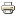# Network of CommonsWorking paper
Author/s:
Rahmi Ilkiliç
Issue number:
2008.030
Publisher:
FEEM
Year:
2008
A tragedy of the commons appears when the users of a common resource have incentives to exploit it more than the socially efficient level. We analyze the situation when the tragedy of the commons is embedded in a network of users and sources. Users play a game of extractions, where they decide how much resource to draw from each source they are connected to. We show that if the value of the resource to the users is linear, then each resource exhibits an isolated problem. There exists a unique equilibrium. But when the users have concave values, the network structure matters. The exploitation at each source depends on the centrality of the links connecting the source to the users. The equilibrium is unique and we provide a formula which expresses the quantities at an equilibrium as a function of a network centrality measure. Next we characterize the efficient levels of extractions by users and outflows from sources. Again, the case of linear values can be broken down source by source. For the case of concave values, we provide a graph decomposition which divides the network into regions according to the availability of sources. Then the efficiency problem can be solved region by region.
Developed by Paolo Gittoi